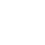# How to create a passage that changes with each visit?

Twine 2.3.14If you are requesting technical assistance with Twine, please specify:Harlowe 3.2.3
Twine Version:2.3.14
Story Format:Harlowe 3.2.3

``````Please can someone advise me?
I have one fairly frequent passage in the game, in which some plot takes place. Only one way out is correct. There I insert a variable (set: \$square it +1).
When the player walks through the passage, I called it the Square, for the first time, it is morning.
When he passes through the square for the second time, it is, for example, noon, and then he goes there in the evening, and so on.
How to write codes so that I can change the plot in one passage?
In the first case I have this:
(if: \$square - 1 < 0)[
Square in the morning.
]
In the second case I have this:
(if: (\$square >= 1))[
Noon Square.
]
I can't think of other ways to write another plot option.
Is it even possible?
Thanks in advance for Jiří Škraba

Please place any example Twine code you provide between three back-ticks
on a separate line before and after the code like this
so it is monospaced and copyable for testing!``````
1 Like

Jiri, please, use the Preformatted text button only for actual code, not for explanations or questions, it would be easier to read you if you do so!

If I understand you correctly, each time the player reaches the passage, the passage should be different. Why making a loop in this case instead of another passage? It might prove easier to set this way.

Anyway, if it’s necessary to make the story come back to the same passage, the problem is your conditions.
Yes, a number is either lesser than 1 or greater or equal to 1.
There’s no way another option could exist. Well, there are the cases where \$square is not defined or not a number, but I’ll suppose you’ve previously defined the variable with a number value.
So you need to set your conditions differently. For instance:

``````(if: \$square is 0)[Square in the morning]
(if: \$square is 1)[Square at noon]
(if: \$square is 2)[Square in the afternoon]
(if: \$square is 3)[Square in the evening]
``````
2 Likes

Hello,

Thank you so much.

That’s exactly it.

I tried it with =, but apparently it cannot be replaced with the = sign.

I’m a beginner and still learning.

Have a nice day

Jiří Škraba1 Like

Indeed an alone ‘=’ means an allocation in many programming languages.
For instance,

``````(set: \$square = 0)
``````

is the same as

``````(set: \$square to 0)
``````
2 Likes

Thanks for the explanation and example.

Jiří Škrába1 Like

As those conditional expressions are mutually exclusive the 2nd and later `(if:)` macro calls should be `(else-if:)` macro calls, which will cause the processing of the set of conditions to stop being evaluated once one of that set evaluates to true.

``````(if: \$square is 0)[Square in the morning]
(else-if: \$square is 1)[Square at noon]
(else-if: \$square is 2)[Square in the afternoon]
(else-if: \$square is 3)[Square in the evening]
``````

And if the maximum numerical range of the `\$square` variable is 0 to 3 then the last of the `(else-if:)` macros could be replaced with an `(else:)` macro, but it’s not required.

``````(if: \$square is 0)[Square in the morning]
(else-if: \$square is 1)[Square at noon]
(else-if: \$square is 2)[Square in the afternoon]
(else:)[Square in the evening]
``````
3 Likes

Hello and thank you.

This works reliably.1 Like